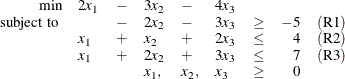## Getting Started: OPTLP Procedure

The following example illustrates how you can use the OPTLP procedure to solve linear programs. Suppose you want to solve the following problem:The corresponding MPS-format SAS data set is as follows:

data example;
input field1 $field2$ field3 $field4 field5$ field6;
datalines;
NAME        .      EXAMPLE   .    .     .
ROWS        .      .         .    .     .
N           COST   .         .    .     .
G           R1     .         .    .     .
L           R2     .         .    .     .
L           R3     .         .    .     .
COLUMNS     .      .         .    .     .
.           X1     COST      2    R2    1
.           X1     R3        1    .     .
.           X2     COST     -3    R1   -2
.           X2     R2        1    R3    2
.           X3     COST     -4    R1   -3
.           X3     R2        2    R3    3
RHS         .      .         .    .     .
.           RHS    R1       -5    R2    4
.           RHS    R3        7    .     .
ENDATA      .      .         .    .     .
;


You can also create this data set from an MPS-format flat file (examp.mps) by using the following SAS macro:

%mps2sasd(mpsfile = "examp.mps", outdata = example);


Note: The SAS macro %MPS2SASD is provided in SAS/OR software. See Converting an MPS/QPS-Format File: %MPS2SASD for details.

You can use the following statement to call the OPTLP procedure:

title1 'The OPTLP Procedure';
proc optlp data = example
objsense  = min
presolver = automatic
algorithm = primal
primalout = expout
dualout   = exdout;
run;


Note: The N designation for COST in the rows section of the data set example also specifies a minimization problem. See the section ROWS Section for details.

The optimal primal and dual solutions are stored in the data sets expout and exdout, respectively, and are displayed in Figure 10.1.

title2 'Primal Solution';
proc print data=expout label;
run;

title2 'Dual Solution';
proc print data=exdout label;
run;


Figure 10.1: Primal and Dual Solution Output

 The OPTLP Procedure Primal Solution

Obs Objective
Function ID
RHS ID Variable
Name
Variable
Type
Objective
Coefficient
Lower
Bound
Upper Bound Variable
Value
Variable
Status
Reduced
Cost
1 COST RHS X1 N 2 0 1.7977E308 0.0 L 2.0
2 COST RHS X2 N -3 0 1.7977E308 2.5 B 0.0
3 COST RHS X3 N -4 0 1.7977E308 0.0 L 0.5
 The OPTLP Procedure Dual Solution

Obs Objective
Function ID
RHS ID Constraint
Name
Constraint
Type
Constraint
RHS
Constraint
Lower
Bound
Constraint
Upper
Bound
Dual Variable
Value
Constraint
Status
Constraint
Activity
1 COST RHS R1 G -5 . . 1.5 U -5.0
2 COST RHS R2 L 4 . . 0.0 B 2.5
3 COST RHS R3 L 7 . . 0.0 B 5.0

For details about the type and status codes displayed for variables and constraints, see the section Data Input and Output.